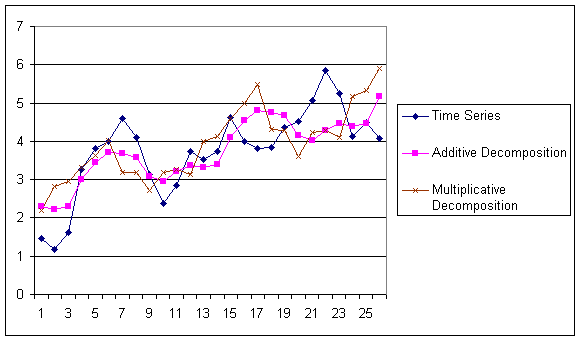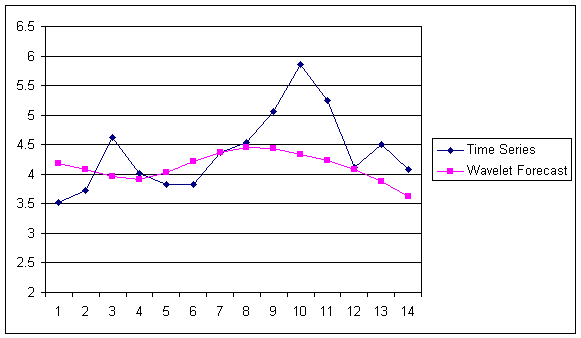# Holt Winter’s, Series Decomposition and Wavelet Benchmarks

How much accuracy shall you expect from time-series forecasting algorithms? As you already know a Mean Absolute Percent Error of 10% can be considered very good but normally this error measure stands in the range 20% to 30%.

To show you how good a forecast produced with IPredict be, we prepared a simple model:

where $\omega$ is a random number with uniform distribution.

this model is periodic and with a linear trend, a situation that is very typical for a lot of businesses and also for the financial markets. On this simple model we calculated a forecast using the three Holt Winter’s methods, the two Series Decomposition methods and IPredict’s unique Wavelet Forecast method. Finally we computed the Mean Absolute Percent Error all the predictions.

The Holt Winter’s predictions look like this:The Series Decomposition predictions look like this:The Wavelet prediction looks like this:As you can see from the two pictures the random noise makes it very difficult to identify correctly the model.

Here you can find also the Mean Absolute Percent Error of this benchmark:

 Holt Winter’s Multiple 10.20% Holt Winter’s Multiplicative 16.60% Holt Winter’s Additive 14.36% Additive Decomposition 14.37% Multiplicative Decomposition 20.08% Wavelet 10.42%

As you can see the best algorithms have a Mean Absolute Percent Error around 10%, a very good result. Less surprisingly the multiplicative models are worse than the additive ones (excluding Wavelet that is neither additive nor multiplicative) since the time series we used is an additive one. The proprietary algorithms are the ones that score better and between them and the best ‘classical’ algorithms there is an outstanding 40% difference.

 Forecasting Methods Holt Winter’s, Series Decomposition and Wavelet Benchmarks Time Series Forecasting Use of the Moving Average in Time-series Forecasting Forecasting Concepts Denoising Techniques Error Statistics Computational Performance Fast Fourier Transform Moving Averages Kernel Smoothing Active Moving Average Savitsky-Golay Smoothing Fractal Projection Downloading Financial Data from Yahoo Multiple Regression Digital Signal Processing Principal Component Analysis Curve Analysis Options Pricing with Black-Scholes Markowitz Optimal Portfolio Time-series preprocessing